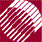ECE532 Biomedical Optics © 1998 Steven L. Jacques, Scott A. Prahl Oregon Graduate Institute

Definitions of variables and arrays

The variables and arrays used by the program are declared here with brief explanations. We shall more fully discuss each parameter as it is used within the program.

/* Propagation parameters */
double	x, y, z;    /* photon position */
double	ux, uy, uz; /* photon trajectory as cosines */
double  uxx, uyy, uzz;	/* temporary values used during SPIN */
double	s;          /* step sizes. s = -log(RND)/mus [cm] */
double	costheta;   /* cos(theta) */
double  sintheta;   /* sin(theta) */
double	cospsi;     /* cos(psi) */
double  sinpsi;     /* sin(psi) */
double	psi;        /* azimuthal angle */
double	i_photon;   /* current photon */
double	W;          /* photon weight */
double	absorb;     /* weighted deposited in a step due to absorption */
short   photon_status;  /* flag = ALIVE=1 or DEAD=0 */

/* other variables */
double	Csph;  /* spherical   photon concentration CC[ir=0..100] */
double	Ccyl;  /* cylindrical photon concentration CC[ir=0..100] */
double	Cpla;  /* planar      photon concentration CC[ir=0..100] */
double	Fsph;       /* fluence in spherical shell */
double	Fcyl;       /* fluence in cylindrical shell */
double	Fpla;       /* fluence in planar shell */
double	mua;        /* absorption coefficient [cm^-1] */
double	mus;        /* scattering coefficient [cm^-1] */
double	g;          /* anisotropy [-] */
double	albedo;     /* albedo of tissue */
double	nt;         /* tissue index of refraction */
double	Nphotons;   /* number of photons in simulation */
short	NR;         /* number of radial positions */
double	r;          /* radial position */
double  dr;         /* radial bin size */
short	ir;         /* index to radial position */
double  shellvolume;  /* volume of shell at radial position r */
double 	CNT;        /* total count of photon weight summed over all bins */

/* dummy variables */
double  rnd;        /* assigned random value 0-1 */
short	i, j;       /* dummy indices */
double	u, temp;    /* dummy variables */
FILE*	target;     /* point to output file */

to next page | Chapter 4 | Course | Home

©1998, Steven L. Jacques, Scott A. Prahl, Oregon Graduate Institute, Oregon Medical Laser Center